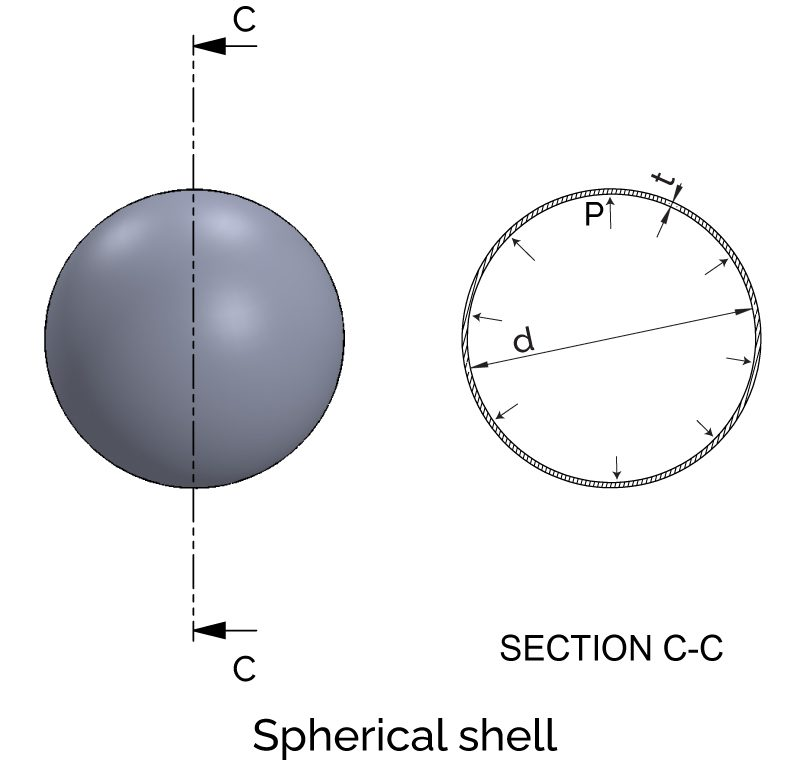# Hoop Stress Calculator

Created by Gabriela Diaz
Last updated: Nov 05, 2022

With this hoop stress calculator, you can expect to easily determine the circumferential stress present in a cylindrical or spherical pressure vessel subjected to internal pressure.

Did you know that hoop stress is also an essential factor in the design of pipelines? Or that the first theoretical analysis of stress in cylindrical boilers took place in the early 19th century? If you didn't, then you're in for a treat! Here we're discussing these and other exciting facts about hoop stress:

• We'll see what hoop stress is;
• The formula to calculate the hoop stress in pressure vessels; and
• How to calculate hoop stress in cylindrical and spherical pressure vessels!

Stay tuned!

## What is hoop stress?

Hoop stress is a critical mechanical parameter in engineering as it provides a way to calculate the resistance of cylindrical and spherical objects subjected to internal pressure. This information is essential in designing and constructing pipelines, pressure vessels, and other structures.

When studying cylindrical objects subjected to internal pressure, as is the case of thin-walled pressure vessels and pipes, we'll encounter two types of stresses:

• Longitudinal stress – stress acting along the direction of the length of the cylinder.
• Hoop o tangential or circumferential stress – stress acting along the tangential direction to the shell's circumference.

During the early 19th century, while conducting steam boilers studies, the engineers William Fairbairn and Eaton Hodgkinson first analyzed the stresses in cylinders, realizing that the hoop stress is double of the longitudinal stress in cylinders.

Because hoop stress has the highest value, it makes it the critical stress, meaning that the maximum permissible stress of the material cannot exceed the circumferential or hoop stress.

💡 With the stress calculator, you can read more about what stress is and how to calculate it!

## Longitudinal and hoop stress formulas - How to calculate hoop stress

Now that you know what hoop stress is let's see how to calculate it. The longitudinal and hoop stress formula uses four variables: diameter, thickness, internal pressure, and joint efficiency. Once you have this information, you can use the respective stress formula to determine its value.

#### Longitudinal and hoop stress formula for cylinders

In the case of cylindrical vessels, the hoop or circumferential stress formula is:

$\small \sigma_h = \cfrac{P d}{2 \, t \,\eta}$

The formula for the longitudinal stress is:

$\small \sigma_l = \cfrac{Pd}{4 \, t \,\eta}$

where:

• $\sigma_h$ – Circumferential or hoop stress;
• $\sigma_l$ – Longitudinal stress;
• $P$ – Internal pressure;
• $d$ – Cylinder or sphere diameter;
• $t$ – Shell thickness; and
• $\eta$ – Joint efficiency.

💡 In the case of pipes, you can get an excellent first approximation using these same equations. Instead of using the outer diameter $d$, you can do the calculations with the mean diameter $d_m$ (mean diameter between inner and outer diameter) if this information is at hand.

#### Hoop stress formula for spheres

For spherical thin-walled pressure vessels, this is the hoop stress or circumferential stress formula:

$\small \sigma_h = \cfrac{P d}{4 \, t \,\eta}$

In the case of spherical structures, the only type of stress element that we find is the hoop stress, meaning there isn't longitudinal stress in spheres:

💡 Notice that for the same diameter $d$ and internal pressure $P$, the hoop stress developed in a spherical thin-walled pressure vessel is half the one that appears in a cylindrical pressure vessel.

In the design and calculations of pressure vessels, other loads are also taken into account, as is the case of the wind load and weight of the vessel itself:

• With the wind load calculator, you can learn what this type of load is and how to determine it.

• If you're designing a vessel for the oil industry, you can use the API gravity calculator to help you determine the weight of the crude oil contained by the vessel.

## How to use the hoop stress calculator

With the hoop stress calculator, you can determine the longitudinal and hoop stress that develops in a spherical or cylindrical thin-walled pressure vessel. To use this tool, just follow these steps:

1. Begin by selecting the shape of the vessel, either Sphere or Cylinder.

2. In the Shell properties section, input the dimensions of the vessel and the value for the internal pressure.

3. If you know the material's properties of the vessel, indicate the Young's modulus (E) and the Poisson's ratio (μ) in the Materials properties section.

4. With this information, the hoop stress calculator will indicate the values for the stresses and the changes in dimensions.

5. That's it! 😮

💡 Because of the operating conditions and internal pressure to which the pressure vessel is subjected, the material can expand or contract, leading to changes in its dimensions.

Gabriela Diaz
Shape
SphereShell properties
in
Diameter of shell (d)
in
Thickness of shell (t)
in
Thickness to diameter ratio (t/d)
Internal pressure (P)
psi
Material properties
Young's modulus (E)
psi
Poisson's ratio (μ)
Stress
Hoop stress (σ_h)
psi
psi
Maximum out of plane shear stress (σ_s2)
psi
Change in dimensions
Change in diameter (𝛿d)
in
Change in volume (𝛿V)
cu in
People also viewed…